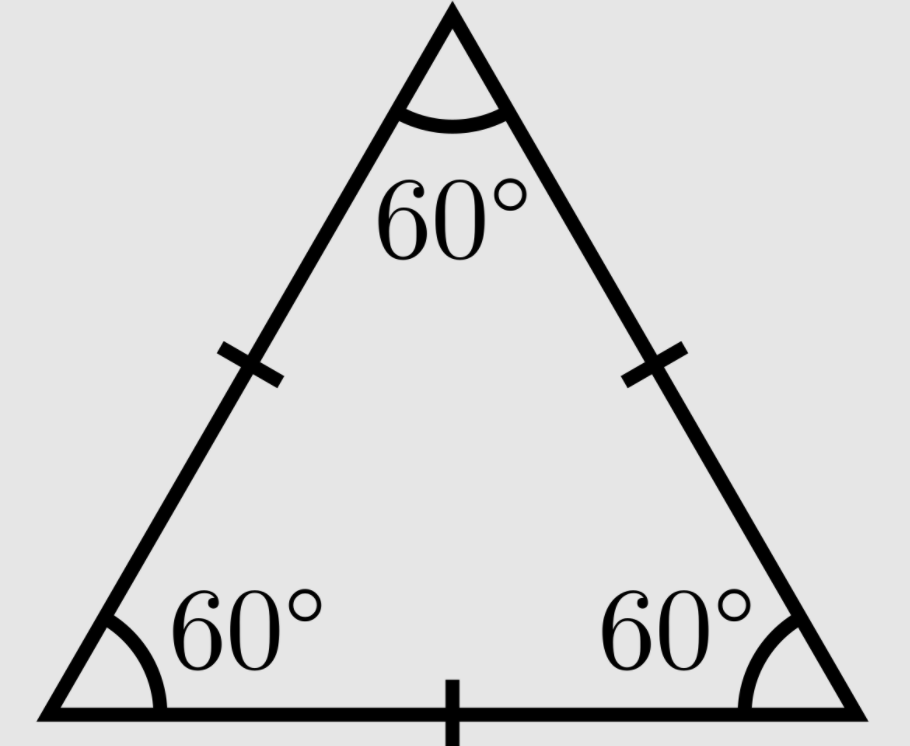100

How many degrees does all 3 angles add up to in a triangle?

180 degrees

100

How many sides does a quadrilateral have?

4

100

What makes a triangle equilateral?

all sides are equal in length

100

What type of angle is this angle?Obtuse

200

What quadrilateral has exactly one set of parallel lines?

What is a trapazoid

200

What is this triangle called?Equilateral Triangle

200

Which is not an attribute of a rectangle?

opposite sides are parallel

opposite sides are equal in length

all sides are equal in length

all angles are right angles

all sides are equal in length

200

True or False: A rhombus has to have all right angles?

False

300

What kind of triangle has 3 sides with different lengths?

What is a scalene triangle

300

What type of angle is 90 degrees?

right angle

300

What is a triangle classified as when it has 2 sides of equal length?

Isosceles
300

Parallel lines cross at a 90 degree angle - True or False?

False

400

A triangle has at least _____ acute angles?

What is 2.

400

A square has one side that is 12 cm long.  What is the PERIMETER of the square?

48 cm

400

True or False? A rhombus has to have all equal sides

True

400

Carrie was 4 ft 11 in tall last year. She grew 6 inches in the past year. How tall is she now?

5 ft 5 in.

600

How many degrees are in a straight angle?

180 degrees

600

A square has one side that is 12 cm long.  What is the AREA of the square?

144 square cm

600

True or False: A square has to have all right angles

True

600

What are line segments that intersect at an angle of 90 degrees

Perpendicular lines

Click to zoom